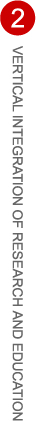# Brent Hawker

## Home

Mentors: Gordan Savin (Spring 2007) & Jingyi Zhu (Fall 2007 & Spring 2008)
Majors: Mathematics, Computer Science, & Economics

Spring 2007 project description:

The equation x^2 - y^2*n = 1 is commonly referred to as Pell's equation. If x and y are integers, and n is a natural number, then a solution (x,y) to this equation is a close rational approximation to the square root of n. This rational approximation can be produced by computing the continued fraction of the square root of n. Using the numerator of the rational approximation as x and the denominator as y solves Pell's equation. As long as n is not a perfect square, the continued fraction expansion can always be computed to solve this equation. However, introducing a variation to Pell's equation, where x^2 - y^2*n = -1, can only be solved for certain values of n. For example, of the first 100 natural numbers, only 20 solve this equation. An interesting property of these numbers is that they contain the set of prime numbers congruent to 1 modulo 4, and none of the prime numbers congruent to 3 modulo 4. This project is a study of continued fractions, and their relation to the solutions of x^2 - y^2*n = -1. It should be possible to determine which values n do and do not solve this equation. For example, it has been shown (see "The Length of the Continued Fraction of P*sqrt(3)" by Michael Lowell Hofmann) that values of the form 3*p^2, where p is a prime number, cannot solve x^2 - y^2*n = -1. If a classification for the values which solve this equation can be made, it may be possible to use continued fractions to predict prime numbers, or gain some insight into the factorization of numbers.

Fall 2007 project description:

This is a study on credit risk which will model the change in credit class of different firms over time. The rating of a company is important because it affects their ability to raise money, and measures the chance that they will default. Markov chains will be used to determine the probability that a firm with a given credit class ends will change credit class or remain the same after a certain period of time. This research will use a reduced-form model as opposed to a discrete structural credit model.

Fall 2007 final report

Spring 2008 project description:

This project will continue the previous research which was performed last semester. A more accurate model to describe the variable nature of credit classes will be researched. The previous semester's research focused on understanding what was going on, and only just began to manipulate data which could be useful as a modeling tool. This research will hopefully provide a useful approximation and more insight into creating an accurate generator matrix. This generator matrix will have a periodic element, and will be used to create a Markov Matrix to diagram the changes in credit classes.

Spring 2008 final report